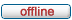forum.alglib.net

ALGLIB forum
 It is currently Wed Oct 16, 2019 10:36 am

 All times are UTC

Forum rules

1. This forum can be used for discussion of both ALGLIB-related and general numerical analysis questions
2. This forum is English-only - postings in other languages will be removed.

 Print view Previous topic | Next topic
Author Message
 Post subject: VBA - Levenberg-MarquardPosted: Fri Apr 17, 2015 4:11 pmJoined: Fri Apr 17, 2015 4:01 pm
Posts: 1
Hi,

I'm a newbie at ALGLIB and also to optimization problems.

I was trying to use Levenberg-Marquardt algortihm with the ALGLIB VBA modules to determine the coeficient of the following expression:

Y=sum(1/X(J)*A), being J=1 to N

I have a bunch of points wiht (A(i),Y(i)) i=1 to M , and I want do determine all the X(J) terms that fit the data.

The following code was written by me (for the first time), to fit the equation with (N=10 and M=401), but somehow it's not working.

Can anyone give me some help!?

Public Const M = 401
Public Const N = 10

Public Sub DemoRoutine()
Dim State As MinLMState
Dim Rep As MinLMReport
Dim S(N - 1) As Double
Dim X(N - 1) As Double

Dim t(M - 1) As Double ' array com as idades : eixo x
Dim t0 As Double ' variavel com idade referencia
t0 = Cells(2, 2)

Dim tau(N - 1) As Double

Dim C(M - 1) As Double 'array com os valores da fun??o compliance C(t,t0) :eixo y

Dim soma_c As Double ' variavel do somat?rio dos valores de C(t,t0)
soma_c = 0

' ler arrays da folha aberta
For I = 0 To M - 1
t(I) = Cells(I + 4, 2) ' gravar em array valores de t() come?am na linha 4, coluna 2
C(I) = Cells(I + 4, 15) 'gravar em array valores de C(t,t0) come?am na linha 4, coluna 15
soma_c = soma_c + C(I) 'soma de todos os valores de C(t,t0) para os intervalo t0 a tfinal
Next

For I = 0 To N - 1
S(I) = (I + 1) ^ 3 'valores inicias da solu??o (formula escolhida por mim)

tau(I) = Cells(2, 3 + I) ' gravar em array valores de tau (i)
Next

Call MinLMCreateFJ(N, M, S, State)
Call MinLMSetCond(State, 0, 0, 0.1, 1000)
Do While MinLMIteration(State)
For I = 0 To N - 1
X(I) = State.X(I)
Cells(I + 4, 16) = X(I)
Next

If State.NeedF Then
State.F = 0
For I = 0 To M - 1
For J = 0 To N - 1
State.F = State.F + (1 / X(J) * (1 - Exp(-(t(I) - t0) / tau(J))))
Next
Next
State.F = Square(State.F - soma_c)

Cells(4, 17) = State.F
End If

If State.NeedFiJ Then
For I = 0 To M - 1
State.FI(I) = 0
Next

For I = 0 To M - 1
For J = 0 To N - 1
State.FI(I) = State.FI(I) + 1 / X(J) * (1 - Exp(-(t(I) - t0) / tau(J)))
Next
State.FI(I) = State.FI(I) - C(I)
Next
For I = 0 To M - 1
For J = 0 To N - 1
State.J(I, J) = -(1 - Exp(-(t(I) - t0) / tau(J))) * 1 / X(J) * 1 / X(J)
Next
Next

End If
Loop
Call MinLMResults(State, S, Rep)

For I = 0 To N - 1
Cells(I + 4, 16) = S(I)
Next
End Sub

TopDisplay posts from previous: All posts1 day7 days2 weeks1 month3 months6 months1 year Sort by AuthorPost timeSubject AscendingDescending

 All times are UTC

Who is online

Users browsing this forum: No registered users and 1 guest

 You cannot post new topics in this forumYou cannot reply to topics in this forumYou cannot edit your posts in this forumYou cannot delete your posts in this forumYou cannot post attachments in this forum

Search for:
 Jump to:  Select a forum ------------------ ALGLIB forum    ALGLIB-discuss
Powered by phpBB © 2000, 2002, 2005, 2007 phpBB Group